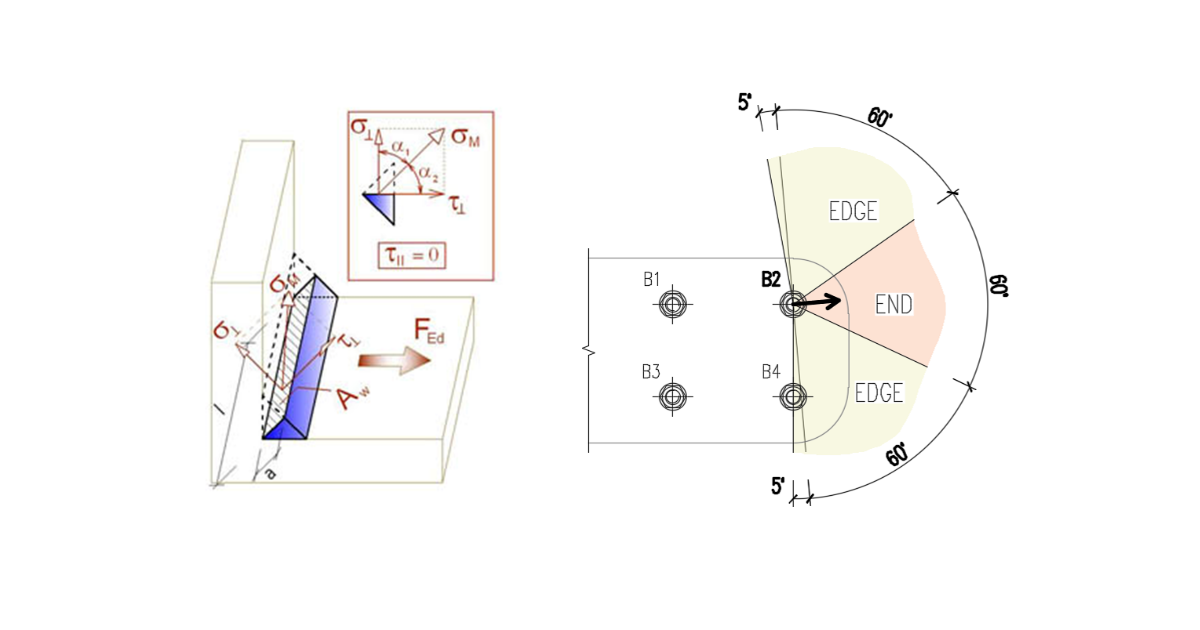### Choose language# Detailing of bolts and welds according to Indian Standard

Detailing of bolt spacing, edge distance, and grip length, weld minimal size, and base plate thickness.
$$BoltsBolt minimum spacing is according to IS 800, Cl. 10.2.2: Centre to centre of the bolt should be larger than $$2.5 \cdot d$$, where $$d$$ is nominal bolt diameter.Minimum end and edge distances measured from the centreline of the bolt are taken according to IS 800, Cl. 10.2.4 as $$1.5 \cdot d_0$$, where $$d_0$$ is the standard hole diameter according to IS 800, Table 19.The grip length of bolts should be limited to $$8d$$ according to IS 800, Cl. 10.3.3.2.WeldsMinimal size of welds is checked according to IS 800, Table 21: Thickness of thicker part [mm]Minimal weld size [mm] $$t \le 10$$3 $$10 < t \le 20$$5 $$20 < t \le 32$$ 6 $$32 < t$$10 Note that weld size is assumed as throat thickness multiplied by $$\sqrt{2}$$.Column baseColumn base thickness should be larger than the thickness of column flange according to IS 800, Cl. 7.4.3.1.$$